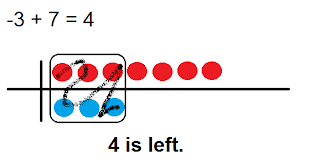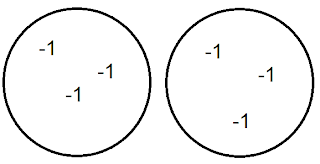## Monday, March 7, 2011

### Carl's Great Big Book of Integers

Chapter 1

What we may remember.
• "When subtracting something that isn't there use a zero pair."
• A zero pair are 2 numbers that have the same value but opposite signs and adds up to zero
• When subtracting or adding integers, you can use a number line or integer chips to help you. When you use chips, generally red symbolizes positive and blue symbolizes negative.
Math Questions :

1) -3 - (-7) = 42) -3 - 7 = -10Chapter 2
Multiplying Integers:

This is what we did in class. (Show example with images)

(+3) x (+2) = 6
(+3) x (-2) = -6
(-3) x (+2) = -6
(-3) x (-2) = 6

Sign Rule:

Even - When you have an even number of negative factors the product is POSITIVE.
Odd - When you have an odd number
of negative factors the product is NEGATIVE.

Chapter 3
Dividing Integers:

Partitive Division (show on number line)

How many groups of +2 are in 6?
6 ÷ 2= 3

-6 ÷ (-2)= 3
How many groups of -2 are in -6?

Quotative Division

Quotative Division is sharing.

(-6) ÷ 2 = -3
Share (-6) with 2 groups.This would be an example of an image of Quotative Division.

Multiplicative Division

-6 ÷ -2 = 3

-3 x -2 = 6
-2 x -3 = 6

-6 ÷ 2 = -3
2 x -3 = -6
-3 x 2 = -6

Chapter 4
Order of Operations with Integers:

This is the order of calculations.

2. Do everything inside brackets first: "( )"
3. Next is to do exponents: x2
4. Then do multiplies and divides from left to right
5. Lastly do the adds and subtracts from left to right

(+5) x (-3) + (-6) ÷ (+3) using B.E.D.M.A.S.

Step 1:

(+5) x (-3) + (-6) ÷ (+3)

Step 2:

[(+5) x (-3) ] + [ (-6) ÷ (+3)]

Step 3:

-15 + -2